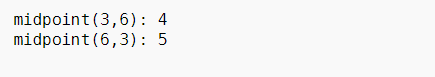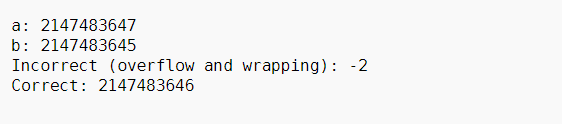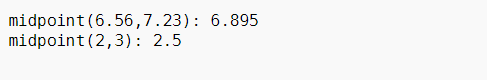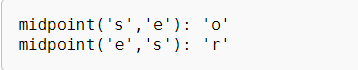Related Articles

# midpoint() in C++20 with Examples

• Last Updated : 04 Feb, 2021

The function midpoint() computes the midpoint of the integers, floating-points, or pointers a and b.

```<numeric>
```

Parameters: This function accepts two data types like integers, floating points, pointer values.

Return:

It returns the midpoints of the given data types.

Alogrithm this function implements:

• Half the sum of a and b without any overflow. Same as, (a + b)/2.
• If a and b are integer types and the sum is odd, the result is rounded towards a.
• If a and b are floating-point types, at most one inexact operation occurs (rounded towards zero).
• If a and b are pointing to x[i] and x[j] respectively of the same array object x (for the purpose of pointer arithmetic), the result will be a pointer to x[i + (j – i)/2] (or, equivalently x[std::midpoint(i, j)]) where the division rounds towards zero. If a and b do not point to elements of the same array object, the behavior is undefined.

Uses Of midpoint() function:

1. Middle point of two integers using midpoint(): Below is the C++ Program to demonstrate the middle point of two integer numbers using midpoint():

## C++

 `// C++ program to demonstrate the``// midpoint function``#include ``#include ``using` `namespace` `std;`` ` `// Driver Code``int` `main()``{``    ``// a and b both of integer type``    ``int` `a = 3;``    ``int` `b = 6;`` ` `    ``cout << ``"midpoint("` `<< a << ``", "``         ``<< b << ``"): "``         ``<< midpoint(a, b) << endl;`` ` `    ``a = 6;``    ``b = 3;``    ``cout << ``"midpoint("` `<< a``         ``<< ``", "` `<< b << ``"): "``         ``<< midpoint(a, b) << endl;`` ` `    ``return` `0;``}`

Output:2. midpoint() can handle cases like an overflow: Below is the C++ program to demonstrate how to handle overflow using midpoint():

## C++

 `// C++ program for the above approach``#include ``#include ``#include ``using` `namespace` `std;`` ` `// Driver Code``int` `main()``{``    ``// a stores maximum storable``    ``// value of integer``    ``int` `a = INT_MAX;`` ` `    ``// b stores maximum storable``    ``// value of integer - 2``    ``int` `b = INT_MAX - 2;`` ` `    ``cout << ``"a: "` `<< a << endl``         ``<< ``"b: "` `<< b << endl``         ``<< ``"Incorrect (overflow"``         ``<< ``" and wrapping): "``         ``<< (a + b) / 2 << endl``         ``<< ``"Correct: "``         ``<< midpoint(a, b) << ``"\n\n"``;`` ` `    ``return` `0;``}`

Output:3. Middle point of two floating number using midpoint(): Below is the C++ program to demonstrate how to find the middle point of two floating-point numbers using midpoint():

## C++

 `// C++ program for the above approach``#include ``#include `` ` `using` `namespace` `std;`` ` `int` `main()``{``    ``// x and y both floating type``    ``float` `x = 6.56;``    ``float` `y = 7.23;`` ` `    ``cout << ``"midpoint("` `<< x``         ``<< ``", "` `<< y << ``"): "``         ``<< midpoint(x, y) << endl;`` ` `    ``x = 2.0;``    ``y = 3.0;`` ` `    ``cout << ``"midpoint("` `<< x``         ``<< ``", "` `<< y << ``"): "``         ``<< midpoint(x, y)``         ``<< endl``         ``<< endl;``}`

Output:4. Middle point between two pointers pointing to the same object using midpoint(): Below is the C++ program to demonstrate how to find the middle point of two pointers pointing to the same object using midpoint():

## C++

 `// C++ program for the above approach``#include ``#include ``using` `namespace` `std;`` ` `// Driver Code``int` `main()``{``    ``// str is a character array``    ``char` `str[] = ``"GeeksforGeeks"``;`` ` `    ``// str1 is pointing to the``    ``// 5-th element in str``    ``char``* str1 = &str;`` ` `    ``// str2 is pointing to the``    ``// 10-th element in str``    ``char``* str2 = &str;`` ` `    ``// str1 and str2 is pointing same``    ``// object str therefore, we can``    ``// logically compute and point a``    ``// middle element in str``    ``cout << ``"midpoint('"` `<< *str1``         ``<< ``"', '"` `<< *str2 << ``"'): '"``         ``<< *midpoint(str1, str2)``         ``<< ``"'"` `<< endl;`` ` `    ``cout << ``"midpoint('"` `<< *str2``         ``<< ``"', '"` `<< *str1 << ``"'): '"``         ``<< *midpoint(str2, str1)``         ``<< ``"'"` `<< endl;``}`

Output:Want to learn from the best curated videos and practice problems, check out the C++ Foundation Course for Basic to Advanced C++ and C++ STL Course for foundation plus STL.  To complete your preparation from learning a language to DS Algo and many more,  please refer Complete Interview Preparation Course.

My Personal Notes arrow_drop_up